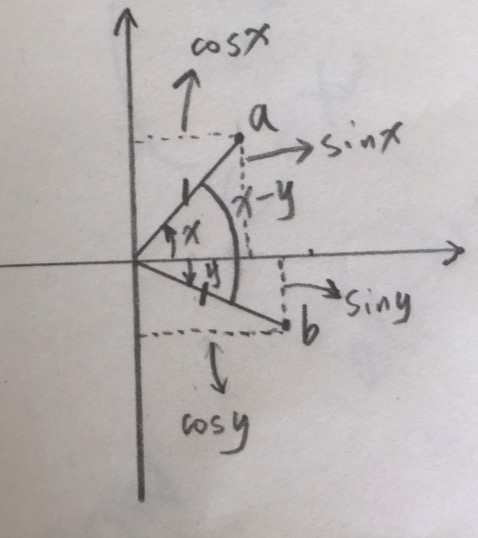## 泰勒展开

### 严谨的推导

$f(x) = g(x) = a_o + a_1x + a_2x^2 + a_3x^3 + … + a_nx^n$

$f(0) = g(0) = a_o$

$f’(0) = g’(0) = a_1$

$f’‘(0) = g’‘(0) = 2a_2$

$f’’‘(0) = g’’‘(0) = 3!a_3$

………

$f^n(0) = g^n(0) = n!a_n$

$a_o=f(0)$

$a_1=\frac{f’(0)}{1!}$

$a_2=\frac{f^2(0)}{2!}$

$a_2=\frac{f^3(0)}{3!}$

……..

$a_n=f^n(0)/n!$

$f(x) = g(x) = f(0) + \frac{f’(0)}{1!}x + \frac{f^2(0)}{2!}x^2 + \frac{f^3(0)}{3!}x^3 + … + \frac{f^n(0)}{n!}x^n$

$f(u) = g(u) = f(u=0) + \frac{f’(u=0)}{1!}x + \frac{f^2(0)}{2!}x^2 + \frac{f^3(0)}{3!}x^3 + … + \frac{f^n(0)}{n!}x^n$

## 欧拉公式

### 先说说虚数

$i$有个特性，挺有意思的，就是用它乘以一个复数，就相当于是这个复数对应的向量，逆时针旋转$90^{\circ}$

### 欧拉公式

\begin{align} e^x=1+x+\frac{x^2}{2!}+\frac{x^3}{3!}+...+\frac{x^n}{n!} \\ cos(x)=1-\frac{x^2}{2!}+\frac{x^4}{4!}-\frac{x^6}{6!}+\frac{x^8}{8!}+... \tag{1} \\ sin(x)=x-\frac{x^3}{3!}+\frac{x^5}{5!}-\frac{x^7}{7!}+\frac{x^9}{9!}+... \tag{2} \\ \end{align}

$e^{i\theta}=1+i\theta+\frac{(i\theta)^2}{2!}+\frac{(i\theta)^3}{3!}+\frac{(i\theta)^4}{4!}+\frac{(i\theta)^5}{5!}+\frac{(i\theta)^6}{6!}+\frac{(i\theta)^7}{7!}+…+\frac{(i\theta)^n}{n!}$

$e^{i\theta}=1+i\theta-\frac{\theta^2}{2!}-\frac{\theta^3}{3!}i+\frac{\theta^4}{4!}+\frac{\theta^5}{5!}i-\frac{\theta^6}{6!}-\frac{\theta^7}{7!}i+…$

$e^{i\theta}=(1-\frac{\theta^2}{2!}+\frac{\theta^4}{4!}-\frac{\theta^6}{6!}+…) + (\theta-\frac{\theta^3}{3!}+\frac{\theta^5}{5!}-\frac{\theta^7}{7!}+…)i$

$$e^{i\theta}=cos(\theta)+sin(\theta)i$$

### 欧拉恒等式

$\color{red}{e^{i\pi}+1=0}$

## 傅里叶变换

• 振幅
• 频率
• 相位

### 三角函数正交性

#### 三角函数积化和差公式$a:(cosx,sinx)$

$b:(cosy,siny)$

$a \cdot b = |a|*|b|*cos(\theta)$

$cos(x-y)=cosx*cosy + sinx*siny$ （1）

$cos(x+y)=cosx*cosy - sinx*siny$ （2）

$sin(x+y)=sinx*cosy + cosx*siny$ （3）

$sin(x-y)=sinx*cosy - cosx*siny$ （4）

（1）和（2）相减，就可以得到，积化和差公式：

$sin(x)*sin(y) = \frac{1}{2}[cos(x-y) - cos(x+y)]$

（3）和（4）相加，就可以得到，积化和差公式：

$sin(x)*cos(y) = \frac{1}{2}[sin(x-y) + sin(x+y)]$

#### 正交性证明

\begin{align} & \int_{-\pi}^\pi sinmx * cosnx dx \\ = & \frac{1}{2}\left[ \int_{-\pi}^\pi sin(n+m)xdx + \int_{-\pi}^\pi sin(n-m)xdx \right] \\ = & -\frac{1}{2}\left[ \frac{1}{n+m} cos(n+m) x|_{-\pi}^\pi + \frac{1}{n+m} cos(n-m) x|_{-\pi}^\pi \right]\\ = & 0 \end{align}

\int_{-\pi}^\pi sinmx * sinnx dx = \left\{ \begin{aligned} 0,&m\neq n \\ \pi,&m=n \neq 0 \end{aligned} \right. \int_{-\pi}^\pi cosmx * cosnx dx = \left\{ \begin{aligned} 0,&m\neq n \\ \pi,&m=n \neq 0 \end{aligned} \right.

### 傅里叶级数

https://zhuanlan.zhihu.com/p/41455378

#### 傅里叶猜想

$f(t)=a_0+\sum_{n=1}^\infty A_n * sin(nt+\phi)$

\begin{align} f(t) & =a_0+\sum_{n=1}^\infty A_n * sin(nt+\phi)\\ & =a_0+\sum_{n=1}^\infty A_n * (sin(\phi) * cos(nt) + cos(\phi) * sin(nt)) \tag{5} \\ & =a_0+\sum_{n=1}^\infty (a_n * sin(\phi)) * cos(nt) + (a_n * cos(\phi) * sin(nt) \\ & =a_0+\sum_{n=1}^\infty a_n * cos(nt) + b_n * sin(nt) \end{align}

$f(t)= a_0 + \sum_{n=1}^\infty a_n * cos(nt) + \sum_{n=1}^\infty b_n * sin(nt)$ (6)

$\int_{-\pi}^{\pi}f(t) dt= \int_{-\pi}^{\pi}a_0 dt + \sum_{n=1}^\infty a_n * \int_{-\pi}^{\pi}cos(nt)dt + \sum_{n=1}^\infty b_n * \int_{-\pi}^{\pi}sin(nt)dt$

$\int_{-\pi}^{\pi}f(t)coskt dt= \sum_{n=1}^\infty a_n * \int_{-\pi}^{\pi}cos(nt)cos(kt)dt$

$\int_{-\pi}^{\pi}f(t)coskt dt= a_k\pi$

$a_k=\frac{1}{\pi}\int_{-\pi}^{\pi}f(t)coskt dt$

$b_k=\frac{1}{\pi}\int_{-\pi}^{\pi}f(t)sinkt dt$